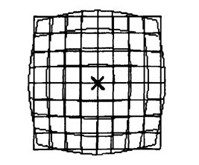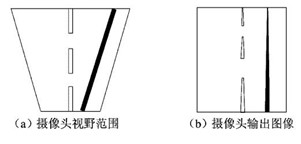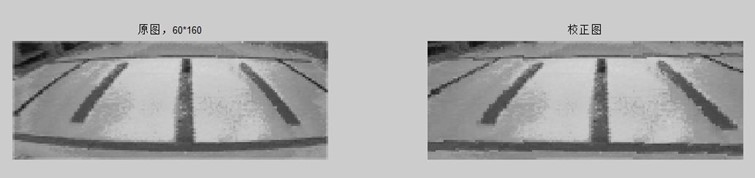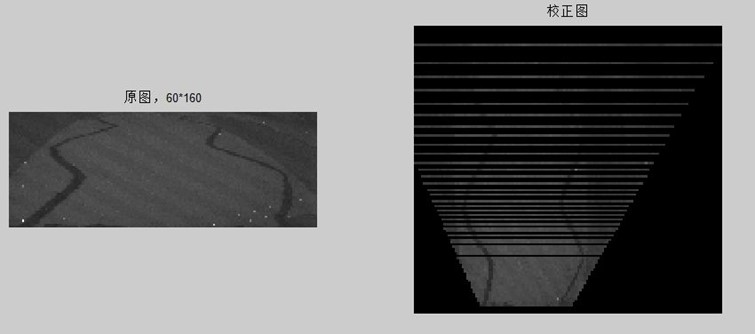# 摄像头图像失真校正

上学的时候玩智能车摄像头组，从发现图像失真、到下载研究各种文档、再到写程序测试、最后实际运用，整个过程很有意思，特别是逆透视变换值得研究。下面的内容是那时候写的MATLAB结课作业，实际运用到K60单片机程序中，是用MATLAB生成图像失真的校正表和反校正表：

//用图像像素定位物理坐标X
const int16 IMG_X[ROW][COL]

//用图像像素定位物理坐标Y

const int16 IMG_Y[ROW][COL]

//物理坐标X轴反校正表  X-列   用物理坐标定位列

const int16 XY_COL[YMAX_1][XMAX2_1]

## 摘要

在机器视觉检测中大视场短焦距摄像机镜头一般都存在一定程度的光学畸变，本文以飞思卡尔智能车大赛为背景，由于摄像头光轴与地面呈一定夹角，于是其成像存在梯形失真;为了扩大视野，广角镜头越来越为很多队伍所采用，于是又存在桶形失真。MATLAB中的数字图像是以矩阵形式表示的,这意味着MATLAB强大的矩阵运算能力用于图像处理非常有利,矩阵运算的语法对MATLAB中的数字图像同样适用。本文继承了图像几何校正法坐标变换的思想精髓，简要论述了MATLAB对摄像头图像畸变失真校正原理和方法。

关键词： MATLAB　摄像头图像 失真校正

## 1、引言

智能车利用摄像头传感器,通过识获取别路面黑色条带状引导线实现自主导航是现阶段智能车较常用的导航方法。由于摄像头镜头为不均匀的透镜，拍摄到的图像会出一定的光学畸变，广角镜头的失真通常会很严重。摄像头所产生的失真主要是桶形失真，如图一。还有摄像头在拍摄图像的时候, 最理想的位置是能垂直于拍摄平面, 这样才能保证图像能按原来的几何比例重现。然而在智能车的实际运用中, 受到智能车车体结构的限制和车体控制需摄像头有一定预瞄距离的要求, 摄像头一般与地平面成一定角度安装。这种角度的存在会造成一定的成像畸变—梯形失真, 图像的畸变会产生一系列问题: 垂直线被拍摄成斜线导致斜率计算错误, 远处的弯道被压缩导致曲率计算错误等等。在这种情况下, 直接利用目标引导线在图像中的相对位置制定控制策略, 会造成较大的误差, 甚至使小车严重偏离导航线。针对此问题, 下面将运用MATLAB分别对桶形失真和梯形失真做校正。## 2、图像校正理论

图像校正是指对失真图像进行的复原性处理。引起图像失真的原因有：成象系统的象差、畸变、带宽有限等造成的图象失真；由于成象器件拍摄姿态和扫描非线性引起的图象几何失真；由于运动模糊、辐射失真、引入噪声等造成的图像失真。图象校正的基本思路是，根据图像失真原因，建立相应的数学模型，从被污染或畸变的图象信号中提取所需要的信息，沿着使图象失真的逆过程恢复图象本来面貌。实际的复原过程是设计一个滤波器，使其能从失真图象中计算得到真实图象的估值，使其根据预先规定的误差准则，最大程度地接近真实图象。从数字图像处理的观点来考察畸变校正, 实际上是一个图像恢复的过程, 是对一幅退化了的图像进行恢复。几何畸变失真主要是表现在图像中像素点发生位移, 从而使图像中物体扭曲变形。造成这类图像退化的原因是多方面的, 而成像系统的非线性, 例如光学系统畸变像差是其中一个主要的因素。

## 3、图像桶形校正

文献一提出的关于几何畸变的校正研究已经比较成熟，其研究思路是：任何一种几何失真都可以用原始图像坐标和畸变图像坐标之间的关系加以描述，因而只需得到原始图像坐标和畸变图像坐标，就可以找出它们之间的对应关系，对图像进行坐标变换，从而得到校正图像。下面运用这种方法在MATLAB对桶形失真做校正。

%% 镜头桶形失真校正(短焦镜头)

img_origin1 = imread('2.2.jpg');

img_origin = rgb2gray(img_origin1);

k1 = -0.000023; % 形变参数，根据实际情况调整

k2 = -0.0000037;

img_size = size( img_origin );

img_undist = zeros( img_size );

img_undist = uint8( img_undist );

for l1 = 1:img_size(1)% 垂直方向

y = l1 - img_size(1)/2;

for l2 = 1:img_size(2)% 水平方向

x = l2 - img_size(2)/2;

x1 = round( x * ( 1 + k1 * x * x + k2 * y * y ) ); %文献一公式

y1 = round( y * ( 1 + k1 * x * x + k2 * y * y ) );

y1 = y1 + img_size(1)/2;

x1 = x1 + img_size(2)/2;

y1 = uint8( y1 );

x1 = uint8( x1 );

img_undist(l1,l2) = img_origin(y1, x1);

end

end

figure(1);

subplot(121); imshow(img_origin);title('原图，60*160');

subplot(122); imshow(img_undist);title('校正图');

imwrite(img_origin,'1.bmp');

imwrite(img_undist,'2.bmp');## 4、图像梯形失真

文献三提出为实现摄像机畸变参数标定, 提出了一种基于非线性逆透视变换模型的标定新方法. 根据线性视变换模型和畸变模型推导出非线性逆透视变换模型, 以及逆透视变换参数与畸变参数之间的关系。下面运用这种方法在MATLAB对梯形失真做校正。

%%首先测量四组物理坐标xy值,生成M矩阵

x1=-20;

y1=20;

u1=13;

v1=37;

x2=20;

y2=20;

u2=126;

v2=37;

x3=-30;

y3=40;

u3=8;

v3=22;

x4=30;

y4=40;

u4=132;

v4=22;

A=[u1,v1,1,0,0,0,-u1*x1,-v1*x1;0,0,0,u1,v1,1,-u1*y1,-v1*y1;u2,v2,1,0,0,0,-u2*x2,-v2*x2;0,0,0,u2,v2,1,-u2*y2,-v2*y2;

u3,v3,1,0,0,0,-u3*x3,-v3*x3;0,0,0,u3,v3,1,-u3*y3,-v3*y3;u4,v4,1,0,0,0,-u4*x4,-v4*x4;0,0,0,u4,v4,1,-u4*y4,-v4*y4];

B=[x1;y1;x2;y2;x3;y3;x4;y4];

M=A\B

M =

1.0477

0.0349

-74.1094

-0.0000

-1.8277

126.8219

-0.0000

0.0530



%% 镜头失真校正--逆变换

clear,clc

img_origin1 = imread('107_1.BMP'); %读取图像文件

img_origin = rgb2gray(img_origin1); %将真彩色图像转换为灰度图像二维矩阵

img_size = size( img_origin ); % 返回矩阵的行数或列数 其中r=size(A,1)该语句返回矩阵A的行数row为垂直方向

m1=1.0477; %上面程序生成的m参数

m2=0.0349;

m3=-74.1094;

m4=0;

m5=-1.8277;

m6=126.8219;

m7=0;

m8=0.0530;

u=160; % 水平方向column

v=0; % 行row为垂直方向

x=(m1*u+m2*v+m3)/(m7*u+m8*v+1);% 水平方向column

y=(m4*u+m5*v+m6)/(m7*u+m8*v+1); % 行row为垂直方向

x=uint16(x);

y=uint16(y); %本段为生成新矩阵容纳校正后的图像

img_undist = zeros(y ,x ); % zeros(m,n)产生m×n的全0矩阵 所有元素初始化为0

new_size = size(img_undist); % 返回m×n全0矩阵的行数或列数

img_undist = uint8( img_undist );

for i = 1:(img_size(1)) % 行row为垂直方向

for j = 1:(img_size(2)) % 水平方向column

u=j; % 水平方向column

v=i; % 垂直方向

x=(m1*u+m2*v+m3)/(m7*u+m8*v+1); % 水平方向column

y=(m4*u+m5*v+m6)/(m7*u+m8*v+1);% 行row为垂直方向

x=int16(x)+new_size(2)/2; %物理坐标用负,现修正显示

y=int16(y);

if(y>0 &&x>0 )

img_undist(y,x)= img_origin(i,j); %对应坐标

end

end

end

img_undist=flipud(img_undist); %矩阵以水平轴翻转

subplot(1,2,1);imshow(img_origin);title('原图，60*160');

subplot(1,2,2);imshow(img_undist);title('校正图');# 5、结束语

MATLAB是一种基于向量而不是标量的高级程序语言，因而MATLAB从本质上就提供了对图像的支持。从图像的数字化过程知道，数字图像实际上就是一组有序的离散数据，使用MATLAB可以结这些离散数据形成的矩阵进行一次整体概念。用MATLAB数字图像处理技术来实现光学系统的畸变校正, 是畸变像差校正的一种新的尝试。它非常适用于那些很难用光学像差校正方法来实现其畸变校正的光学系统。通过对它们所成的畸变图像进行校正处理, 不但能够减小图像的目视失真, 还能够使图像达到测量的要求, 做为定量分析的基础。摄像头失真使其应用受到了很大的限制，本文关于摄像头失真校正的研究，可以应用于任何一个成像系统失真的校正,，对图像识别、图像配准以及图像拼接等研究有很大的帮助，具有较高的实用价值。

[参考文献]

 徐海云 王普 王广生 摄像头失真校正的研究

 甄红涛 齐晓慧 白勇博 智能车摄像头图像畸变矫正的研究

 李颢 杨明 基于非线性逆透视变换的摄像机畸变参数标定